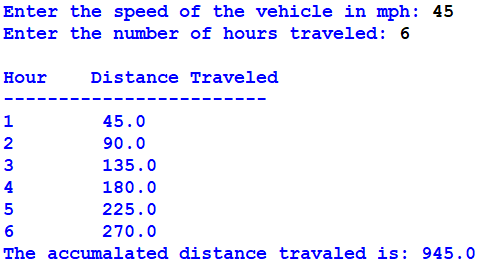## Python program to calculate a vehicle's hourly traveled distance by the given speed and total hours.

The distance a vehicle travels can be calculated as follows:

distance = speed * time

For example, if a train travels 40 miles per hour for three hours, the distance traveled is 120 miles. Write a function that takes the speed of a vehicle and the number of hours it has traveled. It should then use a loop to display the distance the vehicle has traveled for each hour of that time period. It then shows the accumulated distance at

the end. Ask the user for the speed of a vehicle and the number of hours it has traveled.

Here is an example of the desired output:v = int(input("Enter the speed of the vehicle in mph: "))
h = int(input("Enter the number of hours traveled: "))

print("Hour Distance Traveled")
print("----------------------------------")

def table(v, h):
sum = 0
for i in range(1, h+1):
x = float((i) * v)
sum = sum + x
print(i," ",x)
print("The accumalated distance traveled is: ", sum)

table(v, h)